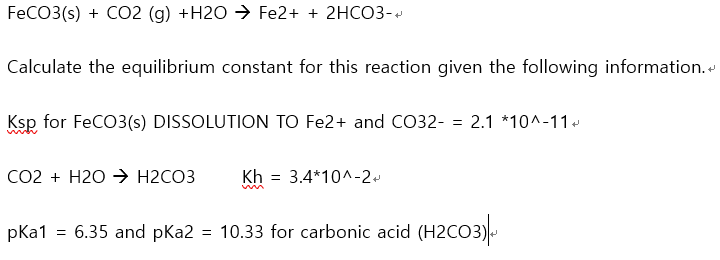# FECO3(s) + CO2 (g) +H2O → Fe2+ + 2HCO3- Calculate the equilibrium constant for this reaction given the following information. Ksp for FeCO3(s) DISSOLUTION TO Fe2+ and CO32- = 2.1 *10^-11. Co2 + H2O > H2CO3 Kh = 3.4*10^-2. pKa1 = 6.35 and pKa2 = 10.33 for carbonic acid (H2CO3)

Questionhelp_outlineImage TranscriptioncloseFECO3(s) + CO2 (g) +H2O → Fe2+ + 2HCO3- Calculate the equilibrium constant for this reaction given the following information. Ksp for FeCO3(s) DISSOLUTION TO Fe2+ and CO32- = 2.1 *10^-11. Co2 + H2O > H2CO3 Kh = 3.4*10^-2. pKa1 = 6.35 and pKa2 = 10.33 for carbonic acid (H2CO3) fullscreen

### Want to see this answer and more?

Experts are waiting 24/7 to provide step-by-step solutions in as fast as 30 minutes!*

*Response times may vary by subject and question complexity. Median response time is 34 minutes for paid subscribers and may be longer for promotional offers.
Tagged in
Science
Chemistry

### Equilibrium Concepts